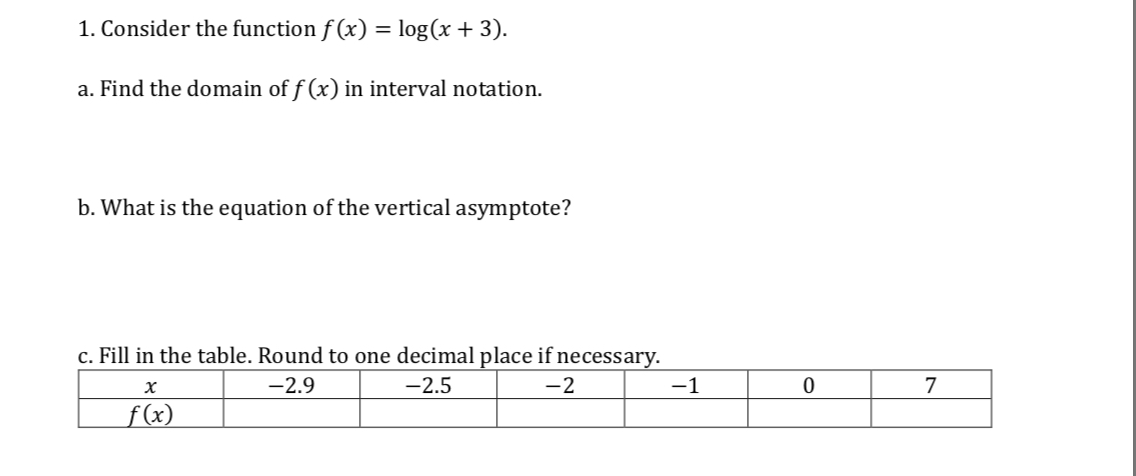I. Consider the function f(x) = log (x + 3)a. Find the domain of f (x) in interval notationb. What is the equation of the vertical asymptote?c. Fill in the table. Round to one decimal place if necessar2.52072.9(x

Questionhelp_outlineImage TranscriptioncloseI. Consider the function f(x) = log (x + 3) a. Find the domain of f (x) in interval notation b. What is the equation of the vertical asymptote? c. Fill in the table. Round to one decimal place if necessar 2.5 2 0 7 2.9 (x fullscreen
Step 1

Definition used:

The domain of a function is the set of all possible values of the independent variable for which the function is defined.

Step 2

Any logarithmic function is defined only for positive values.

Evaluate the domain of log(x+3) as follows.

Step 3

Want to see the full answer?

See Solution

Want to see this answer and more?

Our solutions are written by experts, many with advanced degrees, and available 24/7

See Solution
Tagged in

Logarithms